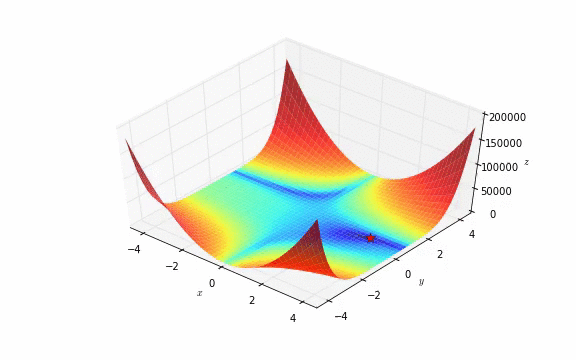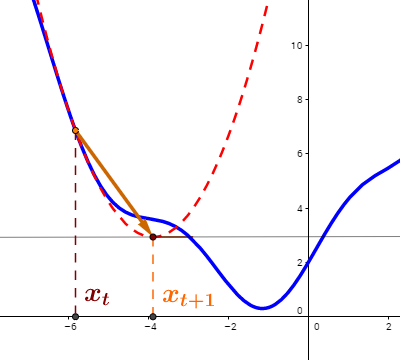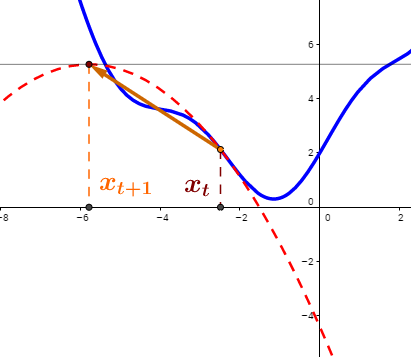### UNCONSTRAINED OPTIMIZATION:

From this simulation:NOTE: For constrained optimization see Lagrange’s method.

#### NEWTON’S ALGORITHM:

From this video by Nando de Freitas, as well as this and this videos by mathematicalmonk on youtube.

It is a method analogous to the Newton’s method to obtaining the roots of a function, as shown in this Geogebra example,

$x_{t+1} = x_t - \frac{f(x_t)}{f'(x_t)}$

but the difference is that for optimization the goal is to find where the derivative is zero, as opposed to the actual function (as in finding the roots). Hence, the formula becomes

$x_{t+1} = x_t - \frac{f'(x_t)}{f''(x_t)}$

which amounts to finding the minima of a quadratic through a second order approximation at a given point:which has the distinct problem that if the function is not convex, we may be headed to a maximum, and the approximation proceeds in the wrong direction:if this is the case, there may be a need to shift to gradient descent.

We want to optimize a scalar-value function of a $$d$$-dimensional vector $$\mathbf\theta$$: $$f(\theta)$$, the loss or objective function.

The gradient vector of $$f(\cdot)$$ with respect to $$\mathbf \theta$$ is the first derivative of a vector-value function:

$\nabla_{\mathbf \theta}f(\mathbf \theta) =\begin{bmatrix} \frac{\partial f(\theta)}{\partial \theta_1}\\ \frac{\partial f(\theta)}{\partial \theta_2}\\ \vdots\\ \frac{\partial f(\theta)}{\partial \theta_n} \end{bmatrix}$

The Hessian matrix is the second derivative of a vector-valued function. The Hessian matrix of $$f(\cdot)$$ with respect to $$\mathbf \theta$$, is defined as

$H=\nabla^2_{\mathbf \theta}f(\mathbf \theta) = \begin{bmatrix} \frac{\partial^2 f(\theta)}{\partial \theta_1^2}& \frac{\partial^2 f(\theta)}{\partial \theta_1 \partial \theta_2}& \cdots& \frac{\partial^2 f(\theta)}{\partial \theta_1\partial\theta_d}\\ \frac{\partial^2 f(\theta)}{\partial \theta_2\partial\theta_1}& \frac{\partial^2 f(\theta)}{\partial \theta_2^2}& \cdots& \frac{\partial^2 f(\theta)}{\partial \theta_2\partial\theta_d}\\ \vdots&\vdots&\ddots&\vdots\\ \frac{\partial^2 f(\theta)}{\partial \theta_d\partial\theta_1}& \frac{\partial^2 f(\theta)}{\partial \theta_d\partial\theta_2}& \cdots& \frac{\partial^2 f(\theta)}{\partial \theta_d\partial\theta_d} \end{bmatrix}$

The most common second-order (second derivative) algorithm is Newton’s algorithm, consisting of weights update of the form:

$\mathbf \theta_{k+1} = \mathbf \theta_k - \mathbf H_k^{-1}\mathbf g_k$

where $$k$$ indexes the steps of the algorithm, and $$\mathbf g_k=\mathbf g(\mathbf \theta_k)$$ is the gradient at step $$k$$. $$\mathbf H^{-1}$$ is the inverse of the Hessian.

This is different than…

$\mathbf \theta_{k+1} = \mathbf \theta_k - \eta_k \mathbf g_k = \mathbf \theta_k - \eta_k \nabla f(\mathbf \theta_k)$

where $$\eta_k$$ is the learning rate at step $$k$$.

Gradient descent is a first order optimization method.

So what is the point of Newton’s algorithm?

Basically, the scalar $$\eta$$ is replaced by a whole matrix $$\mathbf H^{-1}$$. The key is to see that $$\eta$$ needs to be figured out, whereas $$\mathbf H$$, the Hessian, represents the curvature of the function. Because of the negative sign, the steeper the curvature, the slower the updating of the weights, and vice versa.

The formula for the Newton’s algorithm is arrived at by approximating the loss function, $$f(\mathbf \theta)$$, with a second-order Taylor series around $$\mathbf \theta_k$$:

$f_{\text{quad}}(\mathbf \theta)=f(\mathbf \theta_k)+\mathbf g_k^\top (\mathbf \theta - \mathbf \theta_k)+\frac{1}{2}(\mathbf \theta - \mathbf \theta_k)^\top \,\mathbf H_k\,(\mathbf \theta - \mathbf \theta_k)$,

and differentiating to equate it to zero (to get the minima):

$\nabla f_{\text{quad}}(\mathbf \theta)=0+\mathbf g_k + \mathbf H_k (\mathbf \theta - \mathbf \theta_k)=0$

$-\mathbf g_k = \mathbf H_k(\mathbf \theta - \mathbf \theta_k)$

$\mathbf \theta = \mathbf \theta_k - \mathbf H_k^{-1}\mathbf g_k$

This works as long as $$\mathbf H$$ is positive definite.